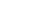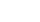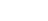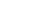xStiffnessEncyclopedia
Stiffness is the resistance of an elastic
Elasticity (physics)
In physics, elasticity is the physical property of a material that returns to its original shape after the stress that made it deform or distort is removed. The relative amount of deformation is called the strain....

body to deformation
Deformation (mechanics)
Deformation in continuum mechanics is the transformation of a body from a reference configuration to a current configuration. A configuration is a set containing the positions of all particles of the body...

by an applied force
Force
In physics, a force is any influence that causes an object to undergo a change in speed, a change in direction, or a change in shape. In other words, a force is that which can cause an object with mass to change its velocity , i.e., to accelerate, or which can cause a flexible object to deform...

along a given degree of freedom (DOF) when a set of loading points and boundary conditions are prescribed on the elastic body.

## Calculations

The stiffness, k, of a body is a measure of the resistance offered by an elastic body to deformation. For an elastic body with a single Degree of Freedom (for example, stretching or compression of a rod), the stiffness is defined aswhere
F is the force applied on the body
δ is the displacement
Displacement field (mechanics)
A displacement field is an assignment of displacement vectors for all points in a region or body that is displaced from one state to another. A displacement vector specifies the position of a point or a particle in reference to an origin or to a previous position...

produced by the force along the same degree of freedom (for instance, the change in length of a stretched spring)

In the International System of Units
International System of Units
The International System of Units is the modern form of the metric system and is generally a system of units of measurement devised around seven base units and the convenience of the number ten. The older metric system included several groups of units...

, stiffness is typically measured in newtons per metre.
In English Units, stiffness is typically measured in pound force (lbf) per inch.

Generally speaking, deflections
Deflection (engineering)
In engineering, deflection is the degree to which a structural element is displaced under a load. It may refer to an angle or a distance.The deflection distance of a member under a load is directly related to the slope of the deflected shape of the member under that load and can be calculated by...

(or motions) of an infinitesimal element (which is viewed as a point) in an elastic body can occur along multiple degrees of freedom (maximum of six DOF at a point). For example, a point on a horizontal beam can undergo both a vertical displacement
Displacement (vector)
A displacement is the shortest distance from the initial to the final position of a point P. Thus, it is the length of an imaginary straight path, typically distinct from the path actually travelled by P...

and a rotation relative to its undeformed axis. When there are M degrees of freedom a M x M matrix
Matrix (mathematics)
In mathematics, a matrix is a rectangular array of numbers, symbols, or expressions. The individual items in a matrix are called its elements or entries. An example of a matrix with six elements isMatrices of the same size can be added or subtracted element by element...

must be used to describe the stiffness at the point. The diagonal terms in the matrix are the direct-related stiffnesses (or simply stiffnesses) along the same degree of freedom and the off-diagonal terms are the coupling stiffnesses between two different degrees of freedom (either at the same or different points) or the same degree of freedom at two different points. In industry, the term influence coefficient is sometimes used to refer to the coupling stiffness.

It is noted that for a body with multiple DOF, the equation above generally does not apply since the applied force generates not only the deflection along its own direction (or degree of freedom), but also those along other directions.

For a body with multiple DOF, in order to calculate a particular direct-related stiffness (the diagonal terms), the corresponding DOF is left free while the remaining should be constrained. Under such a condition, the above equation can be used to obtain the direct-related stiffness for the degree of freedom which is unconstrained. The ratios between the reaction forces (or moments) and the produced deflection are the coupling stiffnesses.

The inverse
Multiplicative inverse
In mathematics, a multiplicative inverse or reciprocal for a number x, denoted by 1/x or x−1, is a number which when multiplied by x yields the multiplicative identity, 1. The multiplicative inverse of a fraction a/b is b/a. For the multiplicative inverse of a real number, divide 1 by the...

of stiffness is compliance, typically measured in units of metres per newton. In rheology it may be defined as the ratio of strain to stress, and so take the units of reciprocal stress, e.g. 1/Pa
Pascal (unit)
The pascal is the SI derived unit of pressure, internal pressure, stress, Young's modulus and tensile strength, named after the French mathematician, physicist, inventor, writer, and philosopher Blaise Pascal. It is a measure of force per unit area, defined as one newton per square metre...

.

## Rotational stiffness

A body may also have a rotational stiffness, k, given bywhere
M is the applied moment
Moment (physics)
In physics, the term moment can refer to many different concepts:*Moment of force is the tendency of a force to twist or rotate an object; see the article torque for details. This is an important, basic concept in engineering and physics. A moment is valued mathematically as the product of the...

θ is the rotation

In the SI system, rotational stiffness is typically measured in newton-metres per radian
Radian is the ratio between the length of an arc and its radius. The radian is the standard unit of angular measure, used in many areas of mathematics. The unit was formerly a SI supplementary unit, but this category was abolished in 1995 and the radian is now considered a SI derived unit...

.

In the SAE system, rotational stiffness is typically measured in inch-pound
Pound-force
The pound force is a unit of force in some systems of measurement including English engineering units and British gravitational units.- Definitions :...

s per degree
Degree (angle)
A degree , usually denoted by ° , is a measurement of plane angle, representing 1⁄360 of a full rotation; one degree is equivalent to π/180 radians...

.

Further measures of stiffness are derived on a similar basis, including:
• shear stiffness - ratio of applied shear
Shear stress
A shear stress, denoted \tau\, , is defined as the component of stress coplanar with a material cross section. Shear stress arises from the force vector component parallel to the cross section...

force to shear deformation
• torsional stiffness - ratio of applied torsion
Torsion (mechanics)
In solid mechanics, torsion is the twisting of an object due to an applied torque. In sections perpendicular to the torque axis, the resultant shear stress in this section is perpendicular to the radius....

moment to angle of twist

## Relationship to elasticity

In general, elastic modulus
Elastic modulus
An elastic modulus, or modulus of elasticity, is the mathematical description of an object or substance's tendency to be deformed elastically when a force is applied to it...

is not the same as stiffness. Elastic modulus is a property of the constituent material; stiffness is a property of a structure. That is, the modulus is an intensive property
Intensive and extensive properties
In the physical sciences, an intensive property , is a physical property of a system that does not depend on the system size or the amount of material in the system: it is scale invariant.By contrast, an extensive property In the physical sciences, an intensive property (also called a bulk...

of the material; stiffness, on the other hand, is an extensive property
Intensive and extensive properties
In the physical sciences, an intensive property , is a physical property of a system that does not depend on the system size or the amount of material in the system: it is scale invariant.By contrast, an extensive property In the physical sciences, an intensive property (also called a bulk...

of the solid body dependent on the material and the shape and boundary conditions. For example, for an element in tension
Tension (mechanics)
In physics, tension is the magnitude of the pulling force exerted by a string, cable, chain, or similar object on another object. It is the opposite of compression. As tension is the magnitude of a force, it is measured in newtons and is always measured parallel to the string on which it applies...

or compression, the axial stiffness iswhere
A is the cross-sectional area,
E is the (tensile) elastic modulus (or Young's modulus
Young's modulus
Young's modulus is a measure of the stiffness of an elastic material and is a quantity used to characterize materials. It is defined as the ratio of the uniaxial stress over the uniaxial strain in the range of stress in which Hooke's Law holds. In solid mechanics, the slope of the stress-strain...

),
L is the length of the element.

Similarly, the rotational stiffness iswhere
"I" is the moment of inertia,
"n" is an integer depending on the boundary condition (=4 for fixed ends)

For the special case of unconstrained uniaxial tension or compression, Young's modulus
Young's modulus
Young's modulus is a measure of the stiffness of an elastic material and is a quantity used to characterize materials. It is defined as the ratio of the uniaxial stress over the uniaxial strain in the range of stress in which Hooke's Law holds. In solid mechanics, the slope of the stress-strain...

can be thought of as a measure of the stiffness of a material.

## Use in engineering

The stiffness of a structure is of principal importance in many engineering applications, so the modulus of elasticity is often one of the primary properties considered when selecting a material. A high modulus of elasticity is sought when deflection
Deflection (engineering)
In engineering, deflection is the degree to which a structural element is displaced under a load. It may refer to an angle or a distance.The deflection distance of a member under a load is directly related to the slope of the deflected shape of the member under that load and can be calculated by...

is undesirable, while a low modulus of elasticity is required when flexibility is needed.

• Elasticity (physics)
Elasticity (physics)
In physics, elasticity is the physical property of a material that returns to its original shape after the stress that made it deform or distort is removed. The relative amount of deformation is called the strain....

• Elastic modulus
Elastic modulus
An elastic modulus, or modulus of elasticity, is the mathematical description of an object or substance's tendency to be deformed elastically when a force is applied to it...

• Mechanical impedance
Mechanical impedance
Mechanical impedance is a measure of how much a structure resists motion when subjected to a given force. It relates forces with velocities acting on a mechanical system. The mechanical impedance of a point on a structure is the ratio of the force applied at a point to the resulting velocity at...

• Hardness
• Hooke's law
Hooke's law
In mechanics, and physics, Hooke's law of elasticity is an approximation that states that the extension of a spring is in direct proportion with the load applied to it. Many materials obey this law as long as the load does not exceed the material's elastic limit. Materials for which Hooke's law...

• Moment of inertia
Moment of inertia
In classical mechanics, moment of inertia, also called mass moment of inertia, rotational inertia, polar moment of inertia of mass, or the angular mass, is a measure of an object's resistance to changes to its rotation. It is the inertia of a rotating body with respect to its rotation...

• Stiffness (mathematics)
• Young's modulus
Young's modulus
Young's modulus is a measure of the stiffness of an elastic material and is a quantity used to characterize materials. It is defined as the ratio of the uniaxial stress over the uniaxial strain in the range of stress in which Hooke's Law holds. In solid mechanics, the slope of the stress-strain...

• Compliant mechanism
Compliant mechanism
In mechanical engineering, compliant mechanisms are flexible mechanisms that transfer an input force or displacement to another point through elastic body deformation...